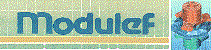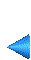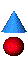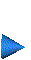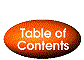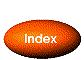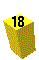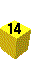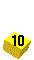## 2.3.3 Definition of the nodes

Generally speaking, modules responsible for the creation and modification of meshes only consider geometrical meshes (i.e. nodes=vertices). For certain finite elements, the nodes may differ from the vertices. Consequently, once the geometric mesh is completed, the non-vertex nodes can be introduced. For example:

• add a node on each edge and indicate that the vertices are nodes (this is the case of a P2-element).
• add a node on each edge and indicate that the vertices are not the nodes (this is the case of a P1-hybrid element).